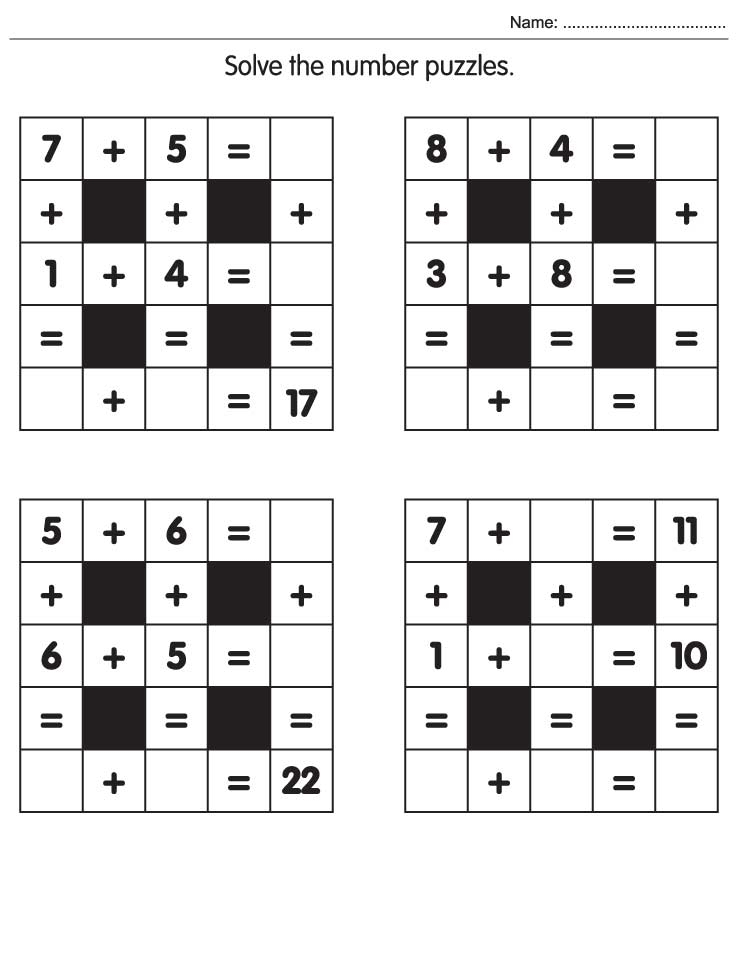# Home

## Worksheet Category

### Solve the number puzzles

Page of 20Page of 20Simple & Easy Maths Worksheets 95Count and write how manyNumber the picture pieces in the correct orderCount and write starsLook at the three sets of animals belowWrite the numbersComplete the sums using the codesSolve the sumsCross out the following as directedDraw a lineCount and writeFollow the table of 8 and join the starsFind the totalColor the pictures that have even numbersJoin the dots from 1-20Mark the numbers which are not in orderEncircle the number 6 and count how many times it appearsColor the given number of objectsDraw, color and complete the sum